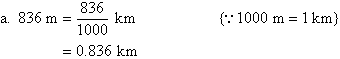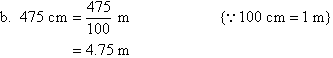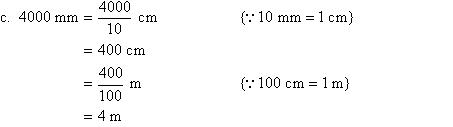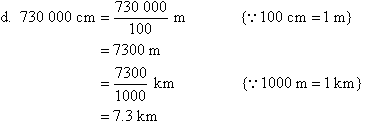Year 7 Interactive Maths - Second Edition

## The Metric System

The metric system was devised during the French Revolution (1789-1799) in order to standardise the different systems of measurement which were then being used.  The metric system has standard units of measurement for problems involving length, area, volume, mass, temperature, electricity and time.  Other units of measurement in the metric system are obtained by multiplying and dividing by powers of 10.

## Measurement of Length

The most common metric units of length used are the kilometre (km), the metre (m), the centimetre (cm) and the millimetre (mm).  These units of length are related as follows: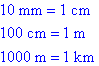Note that we would measure the:

• thickness of the rubber sheet for a table tennis bat in millimetres.
• diagonal of a television screen in centimetres.
• width of a road in metres.
• distance between two airports in kilometres.

Various instruments are used to measure length.  For example:

• rulers and tape measures are marked in millimetres or centimetres to measure shorter lengths accurately.
• a trundle wheel is used to measure length to the nearest metre.  Note that a counter may be attached to the trundle wheel to count metres.
• a car's odometer often measures distance in tenths of a kilometre.

### Converting Metric Units

Consider the lengths of the following lines: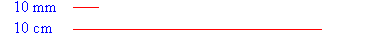It is clear from the visual comparison of the lengths that a centimetre is a larger unit than a millimetre or conversely that a millimetre is a smaller unit than a centimetre.

Likewise, we can say that:

• a metre is a larger unit than a centimetre or conversely that a centimetre is a smaller unit than a metre.
• a kilometre is a larger unit than a metre or conversely that a metre is a smaller unit than a kilometre.

We know that: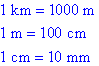###### This suggests that:

To convert length from a large unit into a small unit, multiply by the relevant power of 10.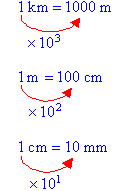To convert length from a small unit into a large unit, divide by the relevant power of 10.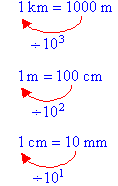#### Example 1

Convert the following measurements.

a.  8 cm to mm
b.  6 m to cm
c.  7 km to m

##### Solution: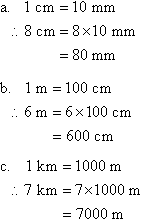#### Example 2

Convert the following measurements.

a.  7 m to mm
b.  5 km to cm
c.  4 km to mm

##### Solution: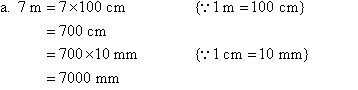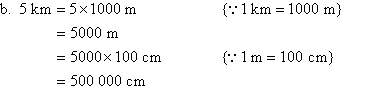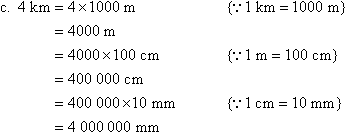#### Example 3

Convert the following measurements.

a.  8.34 cm to mm
b.  38.2 m to cm
c.  6.45 m to mm
d.  3.05 km to mm

##### Solution: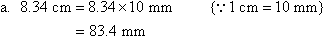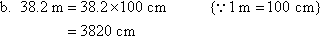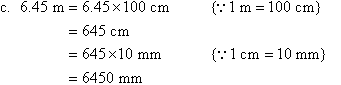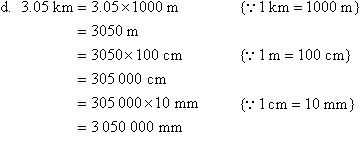#### Example 4

Convert the following measurements.

a.  836 m to km
b.  475 cm to m
c.  4000 mm to m
d.  730 000 cm to km

##### Solution: